# Let's jump in¶

To pull in comma-separated datasets like those for our inflammation data, we make use of a package called numpy. This is the package to use for anything numerical, especially when messing with whole arrays of data.

In :
import numpy

In :
numpy.loadtxt("inflammation-01.csv", delimiter=',')

Out:
array([[ 0.,  0.,  1., ...,  3.,  0.,  0.],
[ 0.,  1.,  2., ...,  1.,  0.,  1.],
[ 0.,  1.,  1., ...,  2.,  1.,  1.],
...,
[ 0.,  1.,  1., ...,  1.,  1.,  1.],
[ 0.,  0.,  0., ...,  0.,  2.,  0.],
[ 0.,  0.,  1., ...,  1.,  1.,  0.]])

We can load one of the inflammation data sets using the numpy.loadtxt function. This function loads the data from disk, but without attaching a name to it, it isn't necessarily stored in memory. A bit about names...

The equal sign is known as the "assignment" operator. For example, given some weight in kg, we can attach a name to that weight like:

In :
weight_kg = 55

In :
print weight_kg

55


The value 55 has the name weight_kg attached to it. To get the value, we need only use the name. We can use this in other expressions that may assign to other names:

In :
weight_lb = 2.2 * weight_kg

In :
weight_lb

Out:
121.00000000000001

weight_lb is calculated from weight_kg. What happens to weight_lb if we change the value attached to weight_kg?

In :
weight_kg = 66

In :
weight_lb

Out:
121.00000000000001

Nothing! That's because weight_lb only remembers the value assigned to it, not how that value was obtained. To update its value, it must be recalculated:

In :
weight_lb = 2.2 * weight_kg

In :
weight_lb

Out:
145.20000000000002

We can assign a name to our data array, which quantifies the inflammation observed in a set of patients (rows) over succeeding days (columns), so we can begin working with it in memory:

In :
data = numpy.loadtxt('inflammation-01.csv', delimiter=',')

In :
print data

[[ 0.  0.  1. ...,  3.  0.  0.]
[ 0.  1.  2. ...,  1.  0.  1.]
[ 0.  1.  1. ...,  2.  1.  1.]
...,
[ 0.  1.  1. ...,  1.  1.  1.]
[ 0.  0.  0. ...,  0.  2.  0.]
[ 0.  0.  1. ...,  1.  1.  0.]]


Arrays are rectangular datasets that consist of all a single data type (in this case, floating-point numbers). They have attributes such as:

In :
data.shape

Out:
(60, 40)

and methods to calculate quantities from their elements such as:

In :
print "mean for all values:", data.mean()

mean for all values: 6.14875


Many of their methods can be fed parameters to change their behavior. For example, instead of the mean of all elements in the array, perhaps we want the mean of each row (that is, for each patient, calculated across all days):

In :
print "mean for each patient:\n",
print data.mean(axis=1)

mean for each patient:
[ 5.45   5.425  6.1    5.9    5.55   6.225  5.975  6.65   6.625  6.525
6.775  5.8    6.225  5.75   5.225  6.3    6.55   5.7    5.85   6.55
5.775  5.825  6.175  6.1    5.8    6.425  6.05   6.025  6.175  6.55
6.175  6.35   6.725  6.125  7.075  5.725  5.925  6.15   6.075  5.75
5.975  5.725  6.3    5.9    6.75   5.925  7.225  6.15   5.95   6.275  5.7
6.1    6.825  5.975  6.725  5.7    6.25   6.4    7.05   5.9  ]


Notice the shape of the output array:

In :
data.mean(axis=1).shape

Out:
(60,)

If you want to know the other parameters a function or method take, put a question mark at the end and run the cell. The documentation will pop up in the notebook:

In :
# displays the docstring
data.mean?


We can also get the mean across all patients for each day:

In :
print "mean for each day:\n",
print data.mean(axis=0)

mean for each day:
[  0.           0.45         1.11666667   1.75         2.43333333   3.15
3.8          3.88333333   5.23333333   5.51666667   5.95         5.9
8.35         7.73333333   8.36666667   9.5          9.58333333
10.63333333  11.56666667  12.35        13.25        11.96666667
11.03333333  10.16666667  10.           8.66666667   9.15         7.25
7.33333333   6.58333333   6.06666667   5.95         5.11666667   3.6
3.3          3.56666667   2.48333333   1.5          1.13333333
0.56666667]


Note: python indices are 0-based, meaning counting goes as 0, 1, 2, 3...; this means that the first axis (rows) is axis 0, the second axis (columns) is axis 1.

How do we get at individual elements in array? We can use indices to grab them. To get the inflammation value for the fifth patient on the 10th day, we can use:

In :
data[4, 9]

Out:
4.0

Remember that python uses 0-based indices! The data for the first patient on the first day is:

In :
data[0, 0]

Out:
0.0

We can select subsets of our data with slicing. To select only the first 5 rows (patients) of the array, we can do:

In :
data[0:5]

Out:
array([[  0.,   0.,   1.,   3.,   1.,   2.,   4.,   7.,   8.,   3.,   3.,
3.,  10.,   5.,   7.,   4.,   7.,   7.,  12.,  18.,   6.,  13.,
11.,  11.,   7.,   7.,   4.,   6.,   8.,   8.,   4.,   4.,   5.,
7.,   3.,   4.,   2.,   3.,   0.,   0.],
[  0.,   1.,   2.,   1.,   2.,   1.,   3.,   2.,   2.,   6.,  10.,
11.,   5.,   9.,   4.,   4.,   7.,  16.,   8.,   6.,  18.,   4.,
12.,   5.,  12.,   7.,  11.,   5.,  11.,   3.,   3.,   5.,   4.,
4.,   5.,   5.,   1.,   1.,   0.,   1.],
[  0.,   1.,   1.,   3.,   3.,   2.,   6.,   2.,   5.,   9.,   5.,
7.,   4.,   5.,   4.,  15.,   5.,  11.,   9.,  10.,  19.,  14.,
12.,  17.,   7.,  12.,  11.,   7.,   4.,   2.,  10.,   5.,   4.,
2.,   2.,   3.,   2.,   2.,   1.,   1.],
[  0.,   0.,   2.,   0.,   4.,   2.,   2.,   1.,   6.,   7.,  10.,
7.,   9.,  13.,   8.,   8.,  15.,  10.,  10.,   7.,  17.,   4.,
4.,   7.,   6.,  15.,   6.,   4.,   9.,  11.,   3.,   5.,   6.,
3.,   3.,   4.,   2.,   3.,   2.,   1.],
[  0.,   1.,   1.,   3.,   3.,   1.,   3.,   5.,   2.,   4.,   4.,
7.,   6.,   5.,   3.,  10.,   8.,  10.,   6.,  17.,   9.,  14.,
9.,   7.,  13.,   9.,  12.,   6.,   7.,   7.,   9.,   6.,   3.,
2.,   2.,   4.,   2.,   0.,   1.,   1.]])
In :
data[0:5].shape

Out:
(5, 40)

This slice should be read as "get the 0th element up to and not including the 5th element". The "not including" is important, and the cause of much initial frustration. It does take some getting used to.

We can also slice the columns; to get only the data for the first five rows (first five patients) and columns 9 through 12 (10th through 13th day), we can use:

In :
data[0:5, 9:13]

Out:
array([[  3.,   3.,   3.,  10.],
[  6.,  10.,  11.,   5.],
[  9.,   5.,   7.,   4.],
[  7.,  10.,   7.,   9.],
[  4.,   4.,   7.,   6.]])

Since the output of a sliced array is another array, we can run methods on these just like before. For example, we can get the standard deviation across days for each patient for this subset:

In :
data[0:5, 9:13].std(axis=1)

Out:
array([ 3.03108891,  2.54950976,  1.92028644,  1.29903811,  1.29903811])

We can treat arrays as if they are single numbers in arithmetic operations. For example, multiplying the array by 2 doubles each element of the array:

In :
double = data * 2

In :
data

Out:
array([[ 0.,  0.,  1., ...,  3.,  0.,  0.],
[ 0.,  1.,  2., ...,  1.,  0.,  1.],
[ 0.,  1.,  1., ...,  2.,  1.,  1.],
...,
[ 0.,  1.,  1., ...,  1.,  1.,  1.],
[ 0.,  0.,  0., ...,  0.,  2.,  0.],
[ 0.,  0.,  1., ...,  1.,  1.,  0.]])
In :
double

Out:
array([[ 0.,  0.,  2., ...,  6.,  0.,  0.],
[ 0.,  2.,  4., ...,  2.,  0.,  2.],
[ 0.,  2.,  2., ...,  4.,  2.,  2.],
...,
[ 0.,  2.,  2., ...,  2.,  2.,  2.],
[ 0.,  0.,  0., ...,  0.,  4.,  0.],
[ 0.,  0.,  2., ...,  2.,  2.,  0.]])

Arithmetic operations can be applied between arrays of the same shape; these operations are performed on corresponding elements (this is also true for multiplication/division).

In :
triple = double + data

In :
triple

Out:
array([[ 0.,  0.,  3., ...,  9.,  0.,  0.],
[ 0.,  3.,  6., ...,  3.,  0.,  3.],
[ 0.,  3.,  3., ...,  6.,  3.,  3.],
...,
[ 0.,  3.,  3., ...,  3.,  3.,  3.],
[ 0.,  0.,  0., ...,  0.,  6.,  0.],
[ 0.,  0.,  3., ...,  3.,  3.,  0.]])

This amounts to $3x_{i,j}^2$, for each element $x_{i,j}$ of the array:

In :
triple * data

Out:
array([[  0.,   0.,   3., ...,  27.,   0.,   0.],
[  0.,   3.,  12., ...,   3.,   0.,   3.],
[  0.,   3.,   3., ...,  12.,   3.,   3.],
...,
[  0.,   3.,   3., ...,   3.,   3.,   3.],
[  0.,   0.,   0., ...,   0.,  12.,   0.],
[  0.,   0.,   3., ...,   3.,   3.,   0.]])

Interested in performing matrix operations between arrays? There are methods built into numpy for that. For example, the inner product:

In :
numpy.inner(triple, data)

Out:
array([[ 5388.,  4266.,  5145., ...,  5802.,  5841.,  5220.],
[ 4266.,  5775.,  5463., ...,  5307.,  5895.,  5019.],
[ 5145.,  5463.,  7182., ...,  6444.,  6930.,  5931.],
...,
[ 5802.,  5307.,  6444., ...,  7320.,  7122.,  6423.],
[ 5841.,  5895.,  6930., ...,  7122.,  8628.,  6771.],
[ 5220.,  5019.,  5931., ...,  6423.,  6771.,  6654.]])

Let's try viewing this array graphically. Just as numpy is the de-facto library for numerically-intensive work, matplotlib is the de-facto library for producing high-quality plots from numerical data. A saying often goes that matplotlib makes easy things easy and hard things possible when it comes to making plots.

In :
import matplotlib.pyplot as plt
%matplotlib inline


We can throw our data into the matplotlib.pyplot.imshow function to view it as a heatmap:

In :
plt.imshow(data)

Out:
<matplotlib.image.AxesImage at 0x7fa6ab0c1590>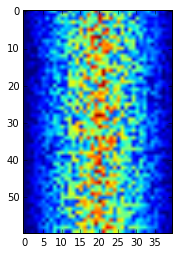We can try viewing the mean inflammation value across patients for each day directly using the matplotlib.pyplot.plot function.

In :
plt.plot(data.mean(axis=0))

Out:
[<matplotlib.lines.Line2D at 0x7fa6aafce310>]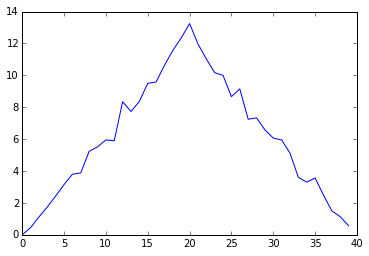Huh...that looks a bit orderly. Almost completely linear with a positive slope to precisely day 20, then back down with about the same slope to day 40...

We can try plotting the max value across all patients for each day:

In :
plt.plot(data.max(axis=0))

Out:
[<matplotlib.lines.Line2D at 0x7fa6a969c550>]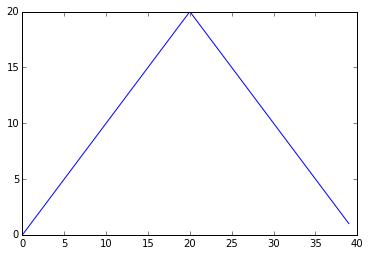That's fishy...

The min across all patients for each day?

In :
plt.plot(data.min(axis=0))

Out:
[<matplotlib.lines.Line2D at 0x7fa6a9650350>]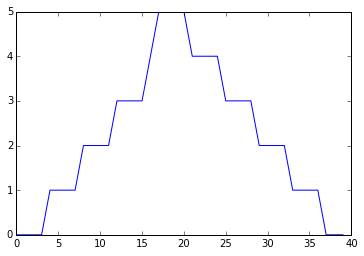A step function, with steps that appear to go in increments of 4 or so across the whole domain of days. Weird...

Matplotlib can make figures that include multiple sub-figures, referred to as axes. We can put the three plots above side-by-side with something like:

In :
# make a figure object
fig = plt.figure(figsize=(10,3))

# make an axis object for the figure, each with a different position
# on a grid that has 1 row and 3 columns of axes

# plot the means
ax1.set_ylabel('average')
ax1.plot(data.mean(axis=0))

# plot the maxes
ax2.set_ylabel('max')
ax2.plot(data.max(axis=0))

# plot the minses
ax3.set_ylabel('min')
ax3.plot(data.min(axis=0))

fig.tight_layout()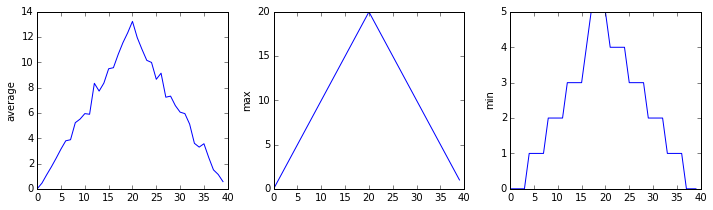It looks like the books were cooked for this data set. What about the other 11? Instead of checking them manually, we can let the computer do what it's good at: doing the same thing repetitively. We need a loop.

# Loops are for looping¶

First, an aside with strings. Most programming languages generally deal in a few basic data types, including integers, floating-point numbers, and characters. A string is a sequence of characters, and we can manipulate them easily with python. For example:

In :
word = 'lead'


We have a string 'lead' that we've assigned the name word. We can examine the string's type, and indeed any object in python, with the type builtin.

In :
print type(word)

<type 'str'>


We can also access its characters (it's a sequence!) with indexing:

In :
word

Out:
'l'
In :
word

Out:
'e'

And slicing:

In :
word[1:]

Out:
'ead'

If I want to output each letter of word on a new line, I could do:

In :
print word
print word
print word
print word

l
e
a
d


But this doesn't scale to words of arbitrary length. Instead, let's let the computer do the boring work of iterating with a for loop:

In :
for letter in word:
print letter

l
e
a
d


This same bit of code will work for any word, including ridiculously long ones. Like this gem:

In :
word = 'supercallifragilisticexpialidocious'
print word

supercallifragilisticexpialidocious

In :
for letter in word:
print letter

s
u
p
e
r
c
a
l
l
i
f
r
a
g
i
l
i
s
t
i
c
e
x
p
i
a
l
i
d
o
c
i
o
u
s


We can do more with loops than iterate through the elements of a sequence. A common use-case is building a sum. For example, getting the number of letters in word:

In :
counter = 0
for letter in word:
counter = counter + 1

print counter

35


For this very particular use, there's a python built-in, which is guaranteed to be more efficient. Use it instead:

In :
len(word)

Out:
35

It works on any sequence! For arrays it gives the length of the first axis, in this case the number of rows:

In :
len(data)

Out:
60

# Lists: ordered collections of other objects (even other lists)¶

Loops work best when they have something to iterate over. In python, the list is a built-in data structure that serves as a container for a sequence of other objects. We can make an empty list with:

In :
stuff = list()

In :
print stuff

[]


And we can append things to the end of the list, in this case a bunch of random strings:

In :
stuff.append('marklar')

In :
stuff.append('chewbacca')

In :
stuff.append('chickenfingers')

In :
stuff

Out:
['marklar', 'chewbacca', 'chickenfingers']

Just like with the word example, we can iterate through the elements of the list:

In :
for item in stuff:
print item

marklar
chewbacca
chickenfingers


And slicing works too! Slicing with negative numbers counts backwards from the end of the list. The slice stuff[-2:] could be read as "get the 2nd-to-last element of stuff, through the last element":

In :
for item in stuff[-2:]:
print item

chewbacca
chickenfingers


So indexing with -1 gives the last element:

In :
stuff[-1]

Out:
'chickenfingers'

And consistently, but uselessly, slicing from and to the same index yeilds nothing. Remember, for a slice n:m, it reads as "get all elements starting with element n and up to but not including element m."

In :
stuff[0:0]

Out:
[]

Lists are mutable: that is, their elements can be changed. For example, we can replace 'chewbacca' with 'hansolo':

In :
stuff

Out:
'chewbacca'
In :
stuff = 'hansolo'

In :
stuff

Out:
'hansolo'

...or replace 'hansolo' with our data array:

In :
stuff = data

In :
stuff

Out:
array([[ 0.,  0.,  1., ...,  3.,  0.,  0.],
[ 0.,  1.,  2., ...,  1.,  0.,  1.],
[ 0.,  1.,  1., ...,  2.,  1.,  1.],
...,
[ 0.,  1.,  1., ...,  1.,  1.,  1.],
[ 0.,  0.,  0., ...,  0.,  2.,  0.],
[ 0.,  0.,  1., ...,  1.,  1.,  0.]])
In :
print stuff

['marklar', array([[ 0.,  0.,  1., ...,  3.,  0.,  0.],
[ 0.,  1.,  2., ...,  1.,  0.,  1.],
[ 0.,  1.,  1., ...,  2.,  1.,  1.],
...,
[ 0.,  1.,  1., ...,  1.,  1.,  1.],
[ 0.,  0.,  0., ...,  0.,  2.,  0.],
[ 0.,  0.,  1., ...,  1.,  1.,  0.]]), 'chickenfingers']


We can make another list, including stuff as an element:

In :
morestuff = ['logs', stuff]

In :
morestuff

Out:
['logs', ['marklar', array([[ 0.,  0.,  1., ...,  3.,  0.,  0.],
[ 0.,  1.,  2., ...,  1.,  0.,  1.],
[ 0.,  1.,  1., ...,  2.,  1.,  1.],
...,
[ 0.,  1.,  1., ...,  1.,  1.,  1.],
[ 0.,  0.,  0., ...,  0.,  2.,  0.],
[ 0.,  0.,  1., ...,  1.,  1.,  0.]]), 'chickenfingers']]

Lists can contain lists can contain lists can contain lists...and of course any number of other python objects. Even functions can be included as elements:

In :
morestuff.append(len)

In :
morestuff

Out:
['logs', ['marklar', array([[ 0.,  0.,  1., ...,  3.,  0.,  0.],
[ 0.,  1.,  2., ...,  1.,  0.,  1.],
[ 0.,  1.,  1., ...,  2.,  1.,  1.],
...,
[ 0.,  1.,  1., ...,  1.,  1.,  1.],
[ 0.,  0.,  0., ...,  0.,  2.,  0.],
[ 0.,  0.,  1., ...,  1.,  1.,  0.]]), 'chickenfingers'], <function len>]

Want the third element of the second element of morestuff?

In :
morestuff

Out:
'chickenfingers'

Lists also have their own methods, which usually alter the list itself:

In :
stuff

Out:
['marklar', array([[ 0.,  0.,  1., ...,  3.,  0.,  0.],
[ 0.,  1.,  2., ...,  1.,  0.,  1.],
[ 0.,  1.,  1., ...,  2.,  1.,  1.],
...,
[ 0.,  1.,  1., ...,  1.,  1.,  1.],
[ 0.,  0.,  0., ...,  0.,  2.,  0.],
[ 0.,  0.,  1., ...,  1.,  1.,  0.]]), 'chickenfingers']
In :
stuff.reverse()

In :
stuff

Out:
['chickenfingers', array([[ 0.,  0.,  1., ...,  3.,  0.,  0.],
[ 0.,  1.,  2., ...,  1.,  0.,  1.],
[ 0.,  1.,  1., ...,  2.,  1.,  1.],
...,
[ 0.,  1.,  1., ...,  1.,  1.,  1.],
[ 0.,  0.,  0., ...,  0.,  2.,  0.],
[ 0.,  0.,  1., ...,  1.,  1.,  0.]]), 'marklar']

list.pop removes the element at the given index:

In :
stuff.pop(1)

Out:
array([[ 0.,  0.,  1., ...,  3.,  0.,  0.],
[ 0.,  1.,  2., ...,  1.,  0.,  1.],
[ 0.,  1.,  1., ...,  2.,  1.,  1.],
...,
[ 0.,  1.,  1., ...,  1.,  1.,  1.],
[ 0.,  0.,  0., ...,  0.,  2.,  0.],
[ 0.,  0.,  1., ...,  1.,  1.,  0.]])

...while list.remove removes the first instance of the given element in the list:

In :
stuff.remove('chickenfingers')


So now our list looks like:

In :
stuff

Out:
['marklar']

# Analyzing at scale¶

We noticed what looked like fraudulent data in the first dataset we analyzed. To look at more datasets without manually running our block of plotting code on each, we'll take advantage of loops and lists.

To grab all the filenames for our inflammation data sets, we'll use the glob library. This includes a function (glob.glob) that will return a list of filenames matching a "glob" pattern, which is what we used for matching files in the bash shell.

In :
import glob

In :
# this will get us all the inflammation data files; we don't care about order
print glob.glob('inflammation*.csv')

['inflammation-07.csv', 'inflammation-04.csv', 'inflammation-01.csv', 'inflammation-05.csv', 'inflammation-08.csv', 'inflammation-10.csv', 'inflammation-09.csv', 'inflammation-06.csv', 'inflammation-12.csv', 'inflammation-03.csv', 'inflammation-02.csv', 'inflammation-11.csv']


Stealing our code block for the plots above and putting them in a loop over filenames, we can look at a whole block of figures. We can slice a subset of the filenames to limit the number we plot at one time.

In :
# get filenames
filenames = glob.glob('inflammation*.csv')

# iterate through each filename, perhaps only a slice of them
for filename in filenames[0:7]:
# so we know what we're looking at
print filename

# make a figure object, with 3 axes in 1 row, 3 columns
fig = plt.figure(figsize=(10,3))

# plot means
ax1.set_ylabel('average')
ax1.plot(data.mean(axis=0))

# plot maxes
ax2.set_ylabel('max')
ax2.plot(data.max(axis=0))

# plot minses
ax3.set_ylabel('min')
ax3.plot(data.min(axis=0))

# this is useful for making the plot elements not overlap
fig.tight_layout()

# this will tell matplotlib to draw the figure now, instead
# of letting the notebook wait to draw them all at once when the cell
# finishes executing
plt.show()

print "done"

inflammation-07.csv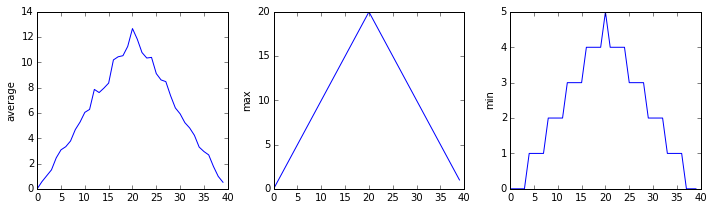inflammation-04.csv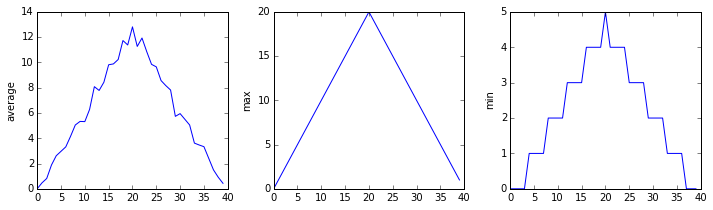inflammation-01.csvinflammation-05.csv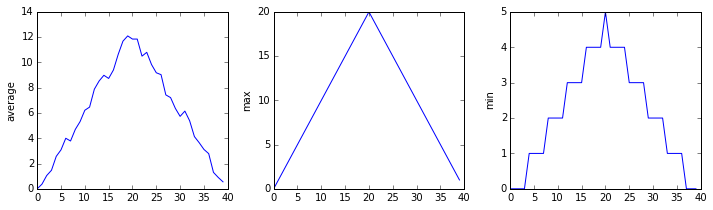inflammation-08.csv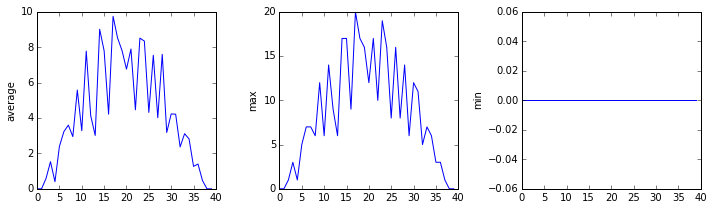inflammation-10.csv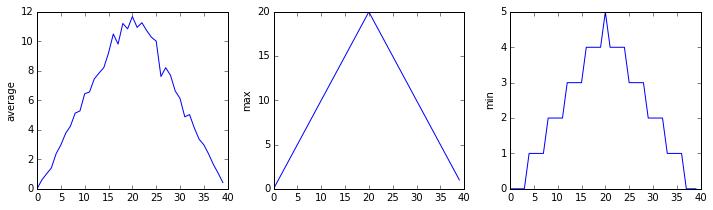inflammation-09.csv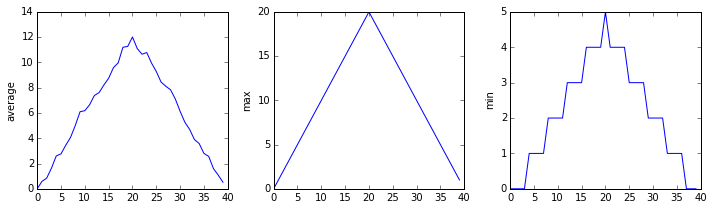done


At least one new pattern emerged: all mins were zero for one dataset! It only gets weirder...

## A short aside: showing off pandas¶

We don't have time to cover pandas in this workshop, but it is quickly becoming the de-facto tool for working with tabular data interactively, and it adds great power to numpy, which it is built on top of. Among its features are the ability to cleanly handle datasets that have missing values (non-rectangular), joining datasets along non-integer indices, grouping datasets by column values, as well as many convenience methods for quickly generating useful visualizations. There's plenty more, but you'll have to explore it on your own for now.

But here's a quick demo; say we want to examine how the distribution of inflammation values of patients changes with each passing day. We can do this easily by putting this data in a pandas.DataFrame, then plotting directly:

In :
import pandas as pd

In :
df = pd.DataFrame(data)


We can look at what the top of our data look like as a DataFrame:

In :
df.head()

Out:
0 1 2 3 4 5 6 7 8 9 ... 30 31 32 33 34 35 36 37 38 39
0 0 0 0 2 4 5 5 4 4 6 ... 3 5 3 1 5 4 4 3 2 1
1 0 1 0 1 3 1 5 3 8 5 ... 8 5 5 4 5 2 2 3 2 1
2 0 1 2 2 4 1 4 2 7 5 ... 2 4 3 7 4 5 3 0 2 1
3 0 1 1 2 2 1 5 3 5 6 ... 3 8 2 4 6 2 2 3 0 1
4 0 1 2 3 3 5 3 4 4 6 ... 9 3 7 1 6 1 3 0 1 1

5 rows × 40 columns

We'll quickly plot a histogram for each day from 1 to 21 (the rise) to see how the distributions shift with day. Cyan distributions are closer to day 0; magenta distributions are closer to day 20.

In :
df.iloc[:, 1:21].plot(kind='hist', legend=False, xlim=(0, 20), bins=range(0, 21, 1), colormap='cool', alpha=.2, histtype='stepfilled', figsize=(13,4))

Out:
<matplotlib.axes._subplots.AxesSubplot at 0x7fa6a9038d50>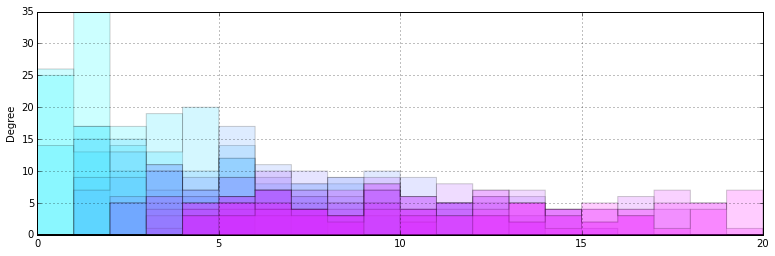We can also plot day 21 through day 39 (the fall) to see how the distributions shift with day. Cyan distributions are closer to day 21; magenta distributions are closer to day 39.

In :
df.iloc[:, 21:40].plot(kind='hist', legend=False, xlim=(0, 20), bins=range(0, 21, 1), colormap='cool', alpha=.2, histtype='stepfilled', figsize=(13,4))

Out:
<matplotlib.axes._subplots.AxesSubplot at 0x7fa6a8b3a0d0>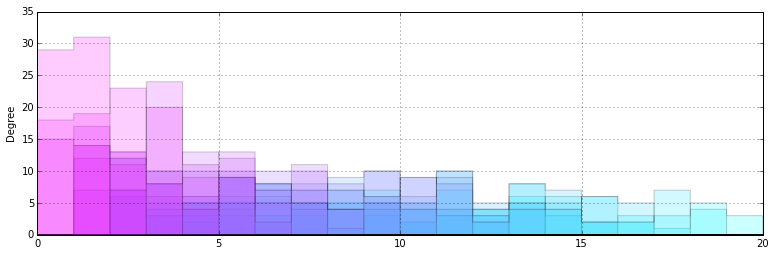We can do the same plots as kernel density estimates quite easily, though with the dataset being discrete and rather small this might not be so useful:

In :
df.iloc[:, 1:21].plot(kind='kde', legend=False, xlim=(0, 20), colormap='cool', figsize=(13,4))
df.iloc[:, 21:41].plot(kind='kde', legend=False, xlim=(0, 20), colormap='cool', figsize=(13,4))

Out:
<matplotlib.axes._subplots.AxesSubplot at 0x7fa6a0193610>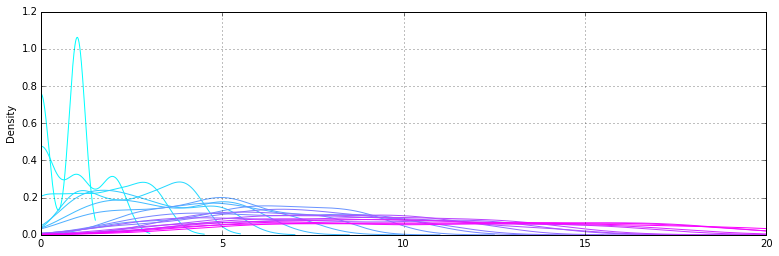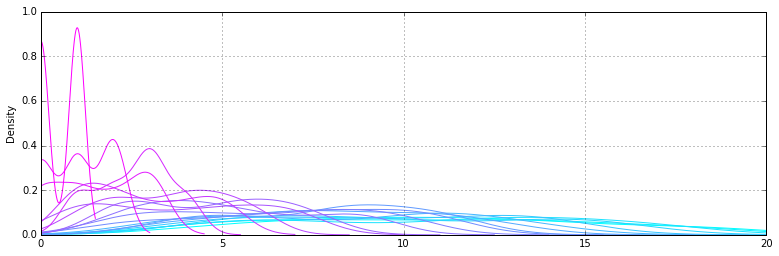Not pretty enough? There are tools for that. seaborn is quite nice, and matplotlib supports style presets:

In :
import seaborn.apionly as sns
plt.style.use('ggplot')
sns.set_style('ticks')

In :
ax = df.iloc[:, 1:21].plot(kind='hist', legend=False, xlim=(0, 20), bins=range(0, 21, 1), colormap='cool', alpha=.2, histtype='stepfilled', figsize=(13,4))
ax.grid(False)
sns.despine(offset=10)
ax = df.iloc[:, 21:40].plot(kind='hist', legend=False, xlim=(0, 20), bins=range(0, 21, 1), colormap='cool', alpha=.2, histtype='stepfilled', figsize=(13,4))
ax.grid(False)
sns.despine(offset=10)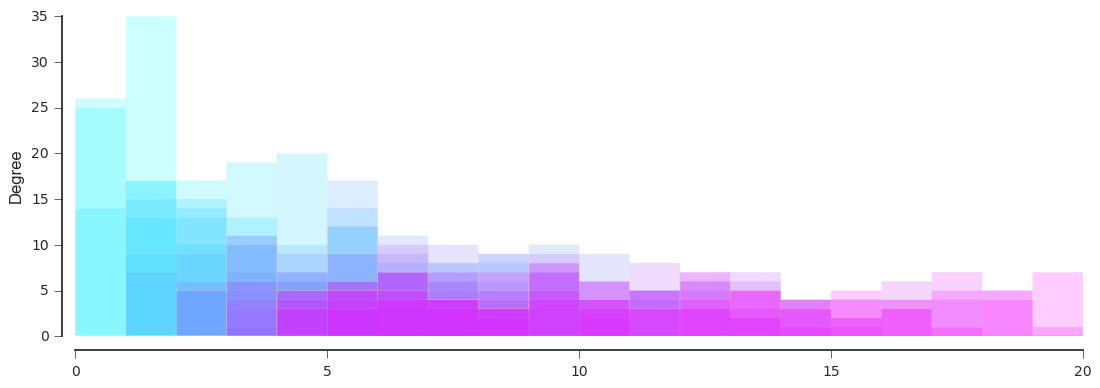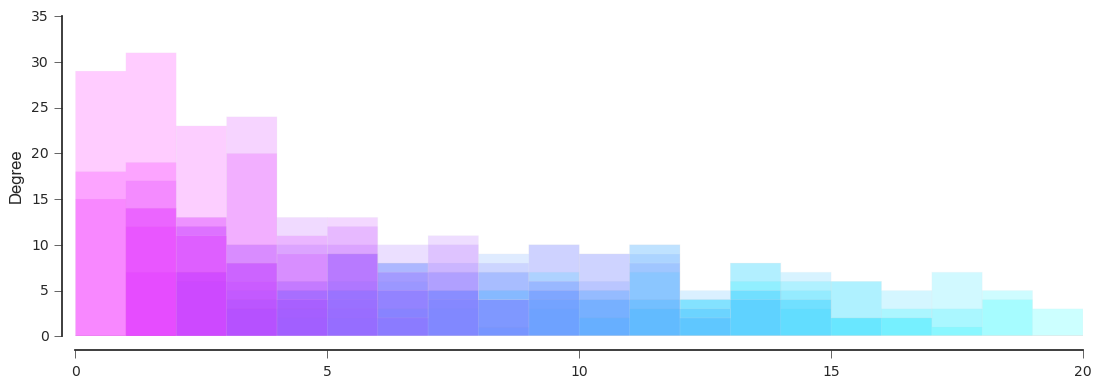# Conditionals: or, what do I do now?¶

We could look at each set of figures for each dataset manually, since this is much easier with the loop we wrote. However, what if we had hundreds of datasets, instead of twelve? That approach wouldn't scale well. Can we write some code to help identify which datasets, and how many, show what flavor of weird behavior?

We can use conditionals. To demonstrate how these work, we'll use absurd toy examples. Say we have some number:

In :
num = 37


We can ask whether that number is greater than 100 with a conditional like this:

In :
if num > 100:
print 'greater'
else:
print 'not greater'

not greater


We could add some more sophisticatioin to the conditional by checking for equality:

In :
if num > 100:
print 'greater'
elif num == 100:
print 'equal!'
else:
print 'not greater'

not greater


...and we could test it:

In :
num = 100

In :
if num > 100:
print 'greater'
elif num == 100:
print 'equal!'
else:
print 'not greater'

equal!


Conditionals can include boolean operators such as and:

In :
if (1 > 0) and (-1 > 0):
print 'both parts are true'
else:
print 'one part is not true'

one part is not true


A "truth table" for and, given two boolean values s1 and s2, each either True or False gives:

s1 s2 s1 and s2
True True True
True False False
False True False
False False False

There's also or:

In :
if (1 > 0) or (-1 > 0):
print 'at least one part is true'
else:
print 'no part is true'

at least one part is true

s1 s2 s1 or s2
True True True
True False True
False True True
False False False

And not:

In :
if (1 > 0) and not (-1 > 0):
print 'both parts are true'
else:
print 'one part is not true'

both parts are true

s1 not s1
True False
False True

## Let's check the data, automatically!¶

We can try and filter the datasets according to some criteria, each matching a case of weird behavior we saw in our plots:

In :
filenames = glob.glob('inflammation*.csv')

# we'll count the number of each weird variety as we go
count_susp_max = 0
count_susp_min = 0
count_okay = 0

for filename in filenames:
print filename

# check for the cases where we see min inflammation as precisely 0 on day 0
# and max inflammation as precisely 20 on day 20
if data.min(axis=0) == 0 and data.max(axis=0) == 20:
print 'suspicious looking maxima!'
# increment count_susp_max
count_susp_max += 1

# check for cases where min is zero across all days
elif data.min(axis=0).sum() == 0:
print 'minima add up to zero!'
# increment count_susp_min
count_susp_min += 1

# keep track of datasets that look okay, at least so far
else:
print "seems 'okay'!"
count_okay += 1

inflammation-07.csv
suspicious looking maxima!
inflammation-04.csv
suspicious looking maxima!
inflammation-01.csv
suspicious looking maxima!
inflammation-05.csv
suspicious looking maxima!
inflammation-08.csv
inflammation-10.csv
suspicious looking maxima!
inflammation-09.csv
suspicious looking maxima!
inflammation-06.csv
suspicious looking maxima!
inflammation-12.csv
suspicious looking maxima!
inflammation-03.csv
inflammation-02.csv
suspicious looking maxima!
inflammation-11.csv


What did we get for each count?

In :
count_susp_max

Out:
9
In :
count_susp_min

Out:
3
In :
count_okay

Out:
0

Sadly, all the data shows fishy behavior. Someone has explaining to do...

# Functions: reusable, modular pieces of code¶

If we had more datasets on the way, we may want to run our tests against these, too. Instead of rewriting the same block of code each time, or copying and pasting it here or there, we should put it into a self-contained function. This way we can also improve it by working on only one piece of code, not a ton of duplicates.

We've already been using functions. One example is the python built-in len:

In :
stuff

Out:
['marklar']
In :
len(stuff)

Out:
1
In :
# run this to view the docstring
len?


Let's write a simple function to show the general form; the function smaller tests whether the input value is smaller than 10, then returns either True or False depending:

In :
# def is followed by the name of the new function, then
# its arguments in parentheses
if somenumber < 10:    # mind the indentation levels!
out = True
else:
out = False

return out             # return gives the value to be output by the function

In :
smaller(157)

Out:
False
In :
smaller(7)

Out:
True
In :
smaller(10)

Out:
False

In fact, for such a simple function it can be condensed greatly:

In :
def smaller(somenumber):


Writing functions make it easier to write better code as well as reusable code. Better in that it is easier to test, and therefore more likely to actually do what you want it to do. Functions can (and should!) be written to do individual tasks (like bash tools) so they can be used as building blocks for larger programs. As an example, let's write a function that calculates the temperature in Kelvin from a temperature in Fahrenheit:

In :
def fahr_to_kelvin(temp):
return ((temp - 32) * (5/9)) + 273.15


Now, let's do some quick sanity tests:

In :
fahr_to_kelvin(32)

Out:
273.15

The freezing point of water looks good!

In :
fahr_to_kelvin(212)

Out:
273.15

Ummm...the boiling point of water should be closer to 373.15 K. What's up? Although languages like python have debugger facilities (see pdb, the built-in debugger), we can debug our simple function by checking the individual pieces to make sure they calculate what we think they should.

In :
print (212 - 32)

180


That works. The next operation?

In :
print (212 - 32) * (5/9)

0


Ah! Something funny is happening here!

In :
180 * (5/9)

Out:
0

This is a common "gotcha" that has to do with how computers store numbers in memory. Arithmetic operations between integers (such as 5 and 9) always yield integers in many programming languages, including python. So when two integers are divided, everything behind the decimal point is truncated (lopped off), leaving behind only what's in front of the decimal point. In this case, just 0.

To fix this, we can convert one of the values into a floating-point number. Division between a float and an integer yields a float:

In :
float(5)/9

Out:
0.5555555555555556

Also we could do this:

In :
5.0/9

Out:
0.5555555555555556

But note, that using float() works even for variables, so it's a bit more useful in general.

We now have:

In :
def fahr_to_kelvin(temp):
return ((temp - 32) * float(5)/9) + 273.15

In :
fahr_to_kelvin(32)

Out:
273.15
In :
fahr_to_kelvin(212)

Out:
373.15

It appears to work!

What about a Kelvin to Celsius converter?

In :
def kelvin_to_celsius(temp):
return temp - 273.15

In :
kelvin_to_celsius(273.15)

Out:
0.0
In :
kelvin_to_celsius(373.15)

Out:
100.0

The sanity checks work out.

We can now write a function to convert fahrenheit to celsius temperatures by composing our two converters together:

In :
def fahrenheit_to_celsius(temp):
temp = fahr_to_kelvin(temp)
temp = kelvin_to_celsius(temp)
return temp


We can make this simpler:

In :
def fahrenheit_to_celsius(temp):
return kelvin_to_celsius(fahr_to_kelvin(temp))


We want to apply the principle of encapsulation (using functions) to our fraud-detection codes. We'll write two functions: one that generates three plots for a dataset given the filename, and another that prints the category of weirdness (or lack thereof) the dataset displays.

In :
import numpy
import matplotlib.pyplot as plt
%matplotlib inline


For the first function, this works:

In :
def make_plots(filename):
# so we know what we're looking at
print filename

# make a figure object, with 3 axes in 1 row, 3 columns
fig = plt.figure(figsize=(10,3))

# plot means
ax1.set_ylabel('average')
ax1.plot(data.mean(axis=0))

# plot maxes
ax2.set_ylabel('max')
ax2.plot(data.max(axis=0))

# plot minses
ax3.set_ylabel('min')
ax3.plot(data.min(axis=0))

# this is useful for making the plot elements not overlap
fig.tight_layout()

plt.show()

In :
make_plots('inflammation-02.csv')

inflammation-02.csv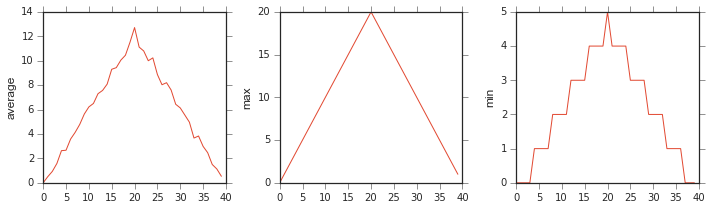And for the second:

In :
def filter_data(filename):
"""Apply labeling criteria for fishy datasets.

Prints the label to standard out

:Arguments:
*filename*
filename for a comma-separated file giving inflammation data

:Returns:
*label*
the flavor of fishiness of this data

"""
print filename

# check for the cases where we see min inflammation as precisely 0 on day 0
# and max inflammation as precisely 20 on day 20
if data.min(axis=0) == 0 and data.max(axis=0) == 20:
out = 'suspicious looking maxima!'

# check for cases where min is zero across all days
elif data.min(axis=0).sum() == 0:
out =  'minima add up to zero!'

# keep track of datasets that look okay, at least so far
else:
out =  "seems 'okay'!"

print out
return out


Note that we've added what looks like documentation in triple quotes (""") at the beginning of the function definition. This allows us to quickly check what our function does in the same way we can for functions like numpy.loadtxt:

In :
filter_data?


In addition to just printing output to stdout, the function also returns the output. This allows us to make use of it, perhaps in more code we would want to write later:

In :
value = filter_data('inflammation-01.csv')

inflammation-01.csv
suspicious looking maxima!

In :
print value

suspicious looking maxima!


Now lets use our functions to examine many files at once, as before!

In :
import glob

In :
filenames = glob.glob('inflammation-*.csv')
filenames

Out:
['inflammation-07.csv',
'inflammation-04.csv',
'inflammation-01.csv',
'inflammation-05.csv',
'inflammation-08.csv',
'inflammation-10.csv',
'inflammation-09.csv',
'inflammation-06.csv',
'inflammation-12.csv',
'inflammation-03.csv',
'inflammation-02.csv',
'inflammation-11.csv']
In :
for f in filenames[:5]:
make_plots(f)
filter_data(f)

inflammation-07.csv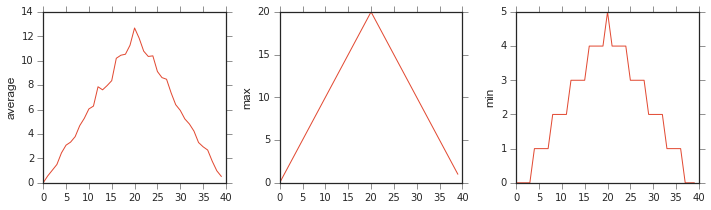inflammation-07.csv
suspicious looking maxima!
inflammation-04.csv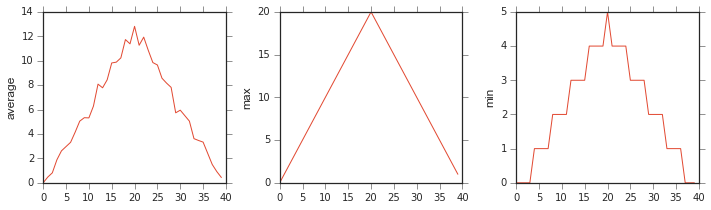inflammation-04.csv
suspicious looking maxima!
inflammation-01.csv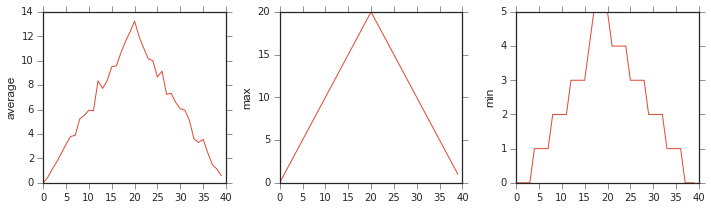inflammation-01.csv
suspicious looking maxima!
inflammation-05.csv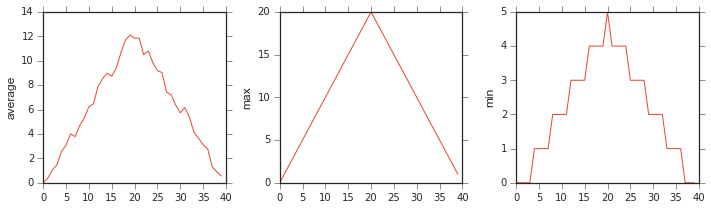inflammation-05.csv
suspicious looking maxima!
inflammation-08.csv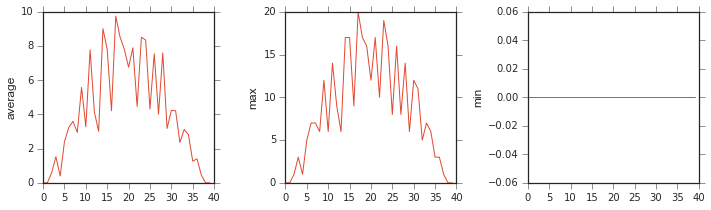inflammation-08.csv


Our for loop went from being long and complicated to simple and easy-to-understand. This is part of the power of functions. Because the human brain can only keep about $7 \pm 2$ concepts in working memory at any given time, functions allow us to abstract and build more complicated code without making it more difficult to understand. Instead of having to worry about how filter_data does its thing, we need only know its inputs and its outputs to wield it effectively. It can be re-used without copying and pasting, and we can maintain it and improve it easily since it can be defined in a single place.

Since we may want to use these functions later in other notebooks/code, we can put their definitions in a file and import it. Opening a text editor and copying the function definitions (and the imports they need) to a file datastuff.py, that looks like this:

In :
%cat datastuff.py

import numpy
import matplotlib.pyplot as plt

def filter_data(filename):
print filename

# check for the cases where we see min inflammation as precisely 0 on day 0
# and max inflammation as precisely 20 on day 20
if data.min(axis=0) == 0 and data.max(axis=0) == 20:
out = 'suspicious looking maxima!'

# check for cases where min is zero across all days
elif data.min(axis=0).sum() == 0:
out =  'minima add up to zero!'

# keep track of datasets that look okay, at least so far
else:
out =  "seems 'okay'!"

print out
return out

def make_plots(filename):
# so we know what we're looking at
print filename

# make a figure object, with 3 axes in 1 row, 3 columns
fig = plt.figure(figsize=(10,3))

# plot means
ax1.set_ylabel('average')
ax1.plot(data.mean(axis=0))

# plot maxes
ax2.set_ylabel('max')
ax2.plot(data.max(axis=0))

# plot minses
ax3.set_ylabel('min')
ax3.plot(data.min(axis=0))

# this is useful for making the plot elements not overlap
fig.tight_layout()

plt.show()


We can then import this as a module:

In :
import datastuff


And use the functions defined inside it:

In :
datastuff.filter_data('inflammation-01.csv')

inflammation-01.csv
suspicious looking maxima!

Out:
'suspicious looking maxima!'
In :
datastuff.make_plots('inflammation-01.csv')

inflammation-01.csvNote that if we make changes to the file and want to see those reflected in our current notebook session, we can use:

In :
reload(datastuff)

Out:
<module 'datastuff' from 'datastuff.pyc'>

# Errors and exceptions¶

Encountering errors is part of coding. If you are coding, you will hit errors. The important thing to remember is that errors that are loud are the best kind, because they usually give hints as to what the problem is. In python, errors are known as exceptions, and there are a few common varieties. Familiarity with these varieties helps to more quickly identify the root of the problem, which means we can more quickly fix it.

Let's import some example code; we need to first add the location of the module we want to import to the list of places python looks for modules:

In :
import sys
sys.path.append('scripts')


Then we can import it:

In :
import errors_01


One example is the IndexError:

In :
errors_01.favorite_ice_cream()

---------------------------------------------------------------------------
IndexError                                Traceback (most recent call last)
<ipython-input-179-44b1b870e6a6> in <module>()
----> 1 errors_01.favorite_ice_cream()

/home/alter/Dropbox/SWC-2015.05.27/osu-data/python/scripts/errors_01.pyc in favorite_ice_cream()
5         "strawberry"
6     ]
----> 7     print ice_creams

IndexError: list index out of range

Let's dissect this. The first line shows where the error originates in our code. In this case it's the only line in the cell. The next set of lines shows where the error originates at the source: that is, inside the definition of errors_01.favorite_ice_cream() in line 7 of the module errors_01. The last line gives us a hint as to the nature of the IndexError: we appear to be using an index for an element of a list that doesn't exist.

We can see this type of error for lists of our own:

In :
a = ['marklar', 'lolcats']

In :
a

---------------------------------------------------------------------------
IndexError                                Traceback (most recent call last)
<ipython-input-181-016a87a854bc> in <module>()
----> 1 a

IndexError: list index out of range

Another common type of exception is the SyntaxError. This is the equivalent of using poor grammar, and the python interpreter is telling us it doesn't understand what we are saying:

In :
for item in a
print item

  File "<ipython-input-182-3804679f8f3a>", line 1
for item in a
^
SyntaxError: invalid syntax


There's also the IOError. "IO" means "input/output", which commonly means reading and writing to disk. It commonly shows up when we try to read a file that doesn't exist:

In :
file_handle = open('myfile.txt', 'r')

---------------------------------------------------------------------------
IOError                                   Traceback (most recent call last)
<ipython-input-184-f6e1ac4aee96> in <module>()
----> 1 file_handle = open('myfile.txt', 'r')

IOError: [Errno 2] No such file or directory: 'myfile.txt'

Or if we try to read a file that we've opened exclusively for writing, in which case python is telling us that our code is probably doing something we don't want it to.

In :
file_handle = open('scripts/myfile.txt', 'w')

In :
file_handle.read()

---------------------------------------------------------------------------
IOError                                   Traceback (most recent call last)
<ipython-input-186-056c6cd01625> in <module>()

IOError: File not open for reading

There are other types of exceptions, but these are the most common. You need not know all of them, but having some knowledge of the basic varieties is valuable for understanding what's going wroing, and then how to fix it!

# Defensive programming: using assertions¶

Say we write a function that does something a bit obtuse (heh): Given the coordinates of the lower-left and upper-right corners of a rectangle, it normalizes the rectangle so that its lower-left coordinate is at the origin (0, 0), and its longest side is 1.0 units long:

In :
def normalize_rectangle(rect):
"""Normalizes a rectangle so that it is at the origin,
and 1.0 units long on its longest axis.

:Arguments:
*rect*
a tuple of size four, giving (x0, y0, x1, y1), the (x,y)
positions of the lower-left and upper-right corner

:Returns:
*newrect*
a tuple of size four, giving the new coordinates of our rectangle's
vertices

"""
x0, y0, x1, y1 = rect

assert x0 < x1, 'Invalid x coordinates'
assert y0 < y1, 'Invalid y coordinates'

dx = x1 - x0
dy = y1 - y0

if dx > dy:
scaled = float(dx) / dy
upper_x, upper_y = 1.0, scaled
else:
scaled = float(dx) / dy
upper_x, upper_y = scaled, 1.0

assert 0 < upper_x <= 1.0, 'Calculated upper X coordinate invalid'
assert 0 < upper_y <= 1.0, 'Calculated upper Y coordinate invalid'

return (0, 0, upper_x, upper_y)


Notice that we added a nice documentation string at the top that explicitly states the functions inputs (arguments) and outputs (returns), so when we use it we don't need to mind its details anymore. Also, at the beginning of the function we have assert statements that check the validity of the inputs, while at the end of the function we have assert statements that check the validity of the outputs.

These statements codify what our documentation says about what the inputs should mean and what the output should look like, guarding against cases where the function is given inputs it wasn't designed to handle.

Let's test the function!

In :
normalize_rectangle((3, 4, 4, 6))

Out:
(0, 0, 0.5, 1.0)

Looks good. First point is at the origin; longest side is 1.0.

In :
normalize_rectangle((0.0, 1.0, 2.0, 5.0))

Out:
(0, 0, 0.5, 1.0)

Nice!

In :
normalize_rectangle((0.0, 0.0, 5.0, 1.0))

---------------------------------------------------------------------------
AssertionError                            Traceback (most recent call last)
<ipython-input-194-a41732464e3f> in <module>()
----> 1 normalize_rectangle((0.0, 0.0, 5.0, 1.0))

<ipython-input-188-57d2a27bd6aa> in normalize_rectangle(rect)
31
32     assert 0 < upper_x <= 1.0, 'Calculated upper X coordinate invalid'
---> 33     assert 0 < upper_y <= 1.0, 'Calculated upper Y coordinate invalid'
34
35     return (0, 0, upper_x, upper_y)

AssertionError: Calculated upper Y coordinate invalid

Oh...what happened here? The inputs should be fine, but an assertion caught something strange in the output. Looking closely at the code, we spot a mistake in line 26:

In :
def normalize_rectangle(rect):
"""Normalizes a rectangle so that it is at the origin,
and 1.0 units long on its longest axis.

:Arguments:
*rect*
a tuple of size four, giving (x0, y0, x1, y1), the (x,y)
positions of the lower-left and upper-right corner

:Returns:
*newrect*
a tuple of size four, giving the new coordinates of our rectangle's
vertices

"""
x0, y0, x1, y1 = rect

assert x0 < x1, 'Invalid x coordinates'
assert y0 < y1, 'Invalid y coordinates'

dx = x1 - x0
dy = y1 - y0

if dx > dy:
scaled = float(dy) / dx
upper_x, upper_y = 1.0, scaled
else:
scaled = float(dx) / dy
upper_x, upper_y = scaled, 1.0

assert 0 < upper_x <= 1.0, 'Calculated upper X coordinate invalid'
assert 0 < upper_y <= 1.0, 'Calculated upper Y coordinate invalid'

return (0, 0, upper_x, upper_y)


And now we get:

In :
normalize_rectangle((0.0, 0.0, 5.0, 1.0))

Out:
(0, 0, 1.0, 0.2)
In :
normalize_rectangle((2, 3, 4, 6))

Out:
(0, 0, 0.6666666666666666, 1.0)

That looks better.

What if we were to try feeding in only three values instead of four?

In :
normalize_rectangle((2, 4, 5))

---------------------------------------------------------------------------
ValueError                                Traceback (most recent call last)
<ipython-input-223-c5d8e9954719> in <module>()
----> 1 normalize_rectangle((2, 4, 5))

<ipython-input-222-d4ce9e9a4cfa> in normalize_rectangle(rect)
14
15     """
---> 16     x0, y0, x1, y1 = rect
17
18     assert x0 < x1, 'Invalid x coordinates'

ValueError: need more than 3 values to unpack

That's a rather unhelpful error message. We can also add an assertion that checks that the input is long enough, and gives a message indicating what the problem is:

In :
def normalize_rectangle(rect):
"""Normalizes a rectangle so that it is at the origin,
and 1.0 units long on its longest axis.

:Arguments:
*rect*
a tuple of size four, giving (x0, y0, x1, y1), the (x,y)
positions of the lower-left and upper-right corner

:Returns:
*newrect*
a tuple of size four, giving the new coordinates of our rectangle's
vertices

"""
assert len(rect) == 4, "Rectangle must have 4 elements"

x0, y0, x1, y1 = rect

assert x0 < x1, 'Invalid x coordinates'
assert y0 < y1, 'Invalid y coordinates'

dx = x1 - x0
dy = y1 - y0

if dx > dy:
scaled = float(dy) / dx
upper_x, upper_y = 1.0, scaled
else:
scaled = float(dx) / dy
upper_x, upper_y = scaled, 1.0

assert 0 < upper_x <= 1.0, 'Calculated upper X coordinate invalid'
assert 0 < upper_y <= 1.0, 'Calculated upper Y coordinate invalid'

return (0, 0, upper_x, upper_y)

In :
normalize_rectangle((2, 4, 5))

---------------------------------------------------------------------------
AssertionError                            Traceback (most recent call last)
<ipython-input-226-c5d8e9954719> in <module>()
----> 1 normalize_rectangle((2, 4, 5))

<ipython-input-225-e9732fa1861f> in normalize_rectangle(rect)
14
15     """
---> 16     assert len(rect) == 4, "Rectangle must have 4 elements"
17
18     x0, y0, x1, y1 = rect

AssertionError: Rectangle must have 4 elements

Now when we use this function six months later, we won't have to scratch our heads too long when this sort of thing happens! Defensive programming in this way makes code more robust, easier to debug, and easier to maintain. This is especially important for scientific code, since incorrect results can occur silently and end up getting published, perhaps only getting uncovered years later. Much embarrassment and misinformation can be avoided by being skeptical of our code, using tools such as assert statements to make sure it keeps doing what we think it should.